# High School Math : Squares

## Example Questions

1 2 4 Next →

### Example Question #1 : How To Find The Length Of The Side Of A Square

The area of square R is 12 times the area of square T. If the area of square R is 48, what is the length of one side of square T?

4

1

16

2

2

Explanation:

We start by dividing the area of square R (48) by 12, to come up with the area of square T, 4.  Then take the square root of the area to get the length of one side, giving us 2.

### Example Question #2 : How To Find The Length Of The Side Of A Square

When the side of a certain square is increased by 2 inches, the area of the resulting square is 64 sq. inches greater than the original square.  What is the length of the side of the original square, in inches?

18

15

14

17

16

15

Explanation:

Let x represent the length of the original square in inches.  Thus the area of the original square is x2.  Two inches are added to x, which is represented by x+2.  The area of the resulting square is (x+2)2.  We are given that the new square is 64 sq. inches greater than the original.  Therefore  we can write the algebraic expression:

x2 + 64 = (x+2)2

FOIL the right side of the equation.

x2 + 64 = x2 + 4x + 4

Subtract xfrom both sides and then continue with the alegbra.

64 = 4x + 4

64 = 4(x + 1)

16 = x + 1

15 = x

Therefore, the length of the original square is 15 inches.

If you plug in the answer choices, you would need to add 2 inches to the value of the answer choice and then take the difference of two squares.  The choice with 15 would be correct because 172 -152 = 64.

### Example Question #3 : How To Find The Length Of The Side Of A Square

If the area of a square is 25 inches squared, what is the perimeter?

25

15

20

10

Not enough information

20

Explanation:

The area of a square is equal to length times width or length squared (since length and width are equal on a square). Therefore, the length of one side is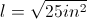or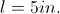The perimeter of a square is the sum of the length of all 4 sides or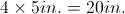### Example Question #4 : How To Find The Length Of The Side Of A Square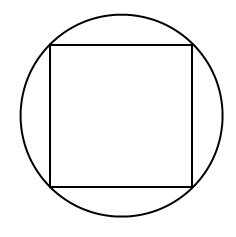A square is inscribed inside a circle, as illustrated above. The radius of the circle is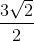units. If all of the square's diagonals pass through the circle's center, what is the area of the square?units squared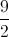units squared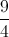units squaredunits squaredunits squaredunits squared

Explanation:

Given that the square's diagonals pass through the circle's center, those diagonals must each form a diameter of the circle. The circle's diameter is twice its radius, i.e.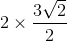, which is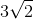. Since this diameter (i.e., the square's diagonal) is the hypotenuse of a right triangle formed by two sides of the square, the length of one of the square's sides can be calculated with the Pythagorean Theorem.replaceandbecause the sides of the square must be equal in length. Since the objective is to solve for the square's area, solve for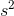since one side squared will be the square's area.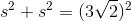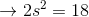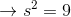units squared

1 2 4 Next →